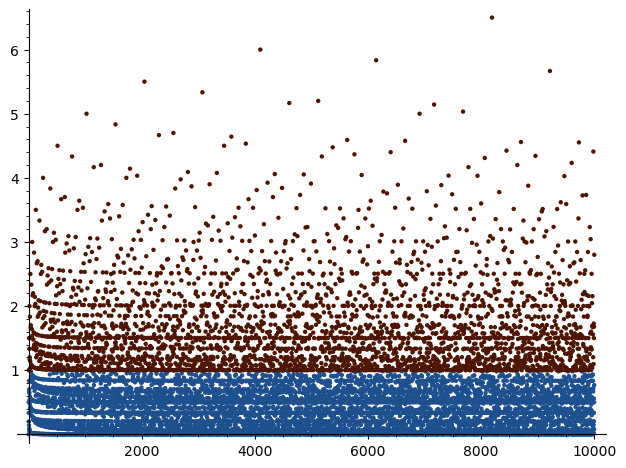Search

# The Arithmetic Derivative (Part 3)

Updated: Oct 15, 2021

## Let us count

The nice thing about the arithmetic derivative is that it takes a natural number to another natural number. This leads to tons of questions about the growth of D(n), such as

1. When is D(n) = n?

2. How often is D(n) < n?

3. What is the significance of the differential equation D(n) = m for different m?

I'll go ahead and answer the first question with no explanation, and we'll talk about it later.

### D(n) = n if and only if n = p^p for prime p

Let's focus on the second question for now. Here are all n < 100 for which D(n) < n.

`1, 2, 3, 5, 6, 7, 9, 10, 11, 13, 14, 15, 17, 19, 21, 22, 23, 25, 26, 29, 31, 33, 34, 35, 37, 38, 39, 41, 42, 43, 45, 46, 47, 49, 50, 51, 53, 55, 57, 58, 59, 61, 62, 63, 65, 66, 67, 69, 70, 71, 73, 74, 75, 77, 78, 79, 82, 83, 85, 86, 87, 89, 91, 93, 94, 95, 97, 98, 99`
• For the first 10 numbers, 80% have D(n) < n.

• For the first 1000 numbers, 68% have D(n) < n.

• For the first 100000 numbers, 68.234% have D(n) < n.

So it seems that MOST numbers get smaller when D operates on them. Testing up to a million, 68.2183% numbers get smaller.This graph shows the area where D(n) < n in blue, which helps explain the 68% number - the points are very densely packed into that area, whereas the red points are more spread out. Another way to view this is to graph D(n)/n, as below.## Starter Differential Equations

The general form of a first-order differential equation would be D(n) = m. If we fix the m, then what can we say about the solutions n?

• If m = 0, then we know that n = 0,1.

• If m = 1, then we know that n = p, a prime number.

• The first actual case: m = 2. If D(n) = 2, then n is not prime, so we can write n = ab, and neither is 1. Then D(n) = aD(b) + D(a)b. If this is equal to 2, then both aD(b) and D(a)b must be equal to 1. But we already know a and b aren't 1, so this is impossible!

Therefore, there are NO solutions to D(n) = 2. This brings a natural question:

Question: For which m does D(n) = m have a solution?

Here are the first few m so that D(n) = m has no solution:

`2, 3, 11, 17, 23, 29, 35, 37, 47, 53, 57, 65, 67, 79, 83, 89, 93, 97, 107, 117, 125, 127, 137, 145, 149, 157, 163, 173, 177, 179`

Or a more general question:

Question: Let S(m) be the set of solutions n such that D(n) = m. What is the size of S(m)?

Let's take our reasoning on m = 2 and expand it a bit. If a,b > 1, then D(a), D(b) >= 1, and

### D(ab) = aD(b) + D(a)b >= a + b

with equality only when a and b are both prime. If we want to maximize (in the usual sense) a + b with the constraint that n = ab, then we substitute b = n/a into a + b, take the derivative with respect to a, set it equal to 0, and solve.

### a = sqrt(n)

Therefore, we find that if n > 1 is not prime, then

### D(n) >= 2sqrt(n)

Often the derivative is MUCH higher. And we know that equality holds only if n = p^2.

This is very helpful as it gives us a bound when solving our differential equations. If we want to solve the equation D(n) = m for a fixed m, then we need m >= 2sqrt(n), meaning we only need to check n <= (m/2)^2. What this guarantees is that S(m) is finite for all m > 1. Specifically,